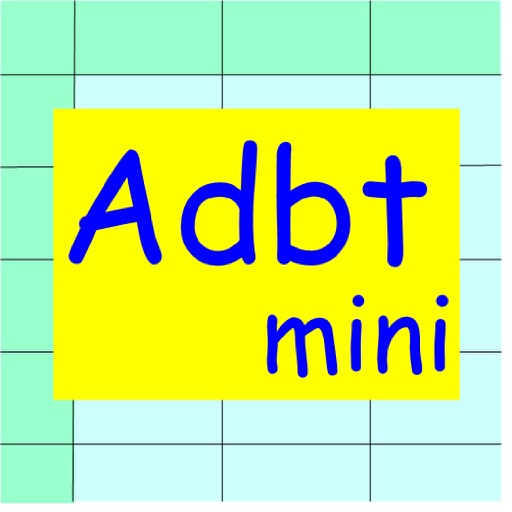## Adiabatic process is a process in which heat exchange does not take place between a　thermodynamic system and its surroundings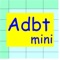by Mineo Yamauchi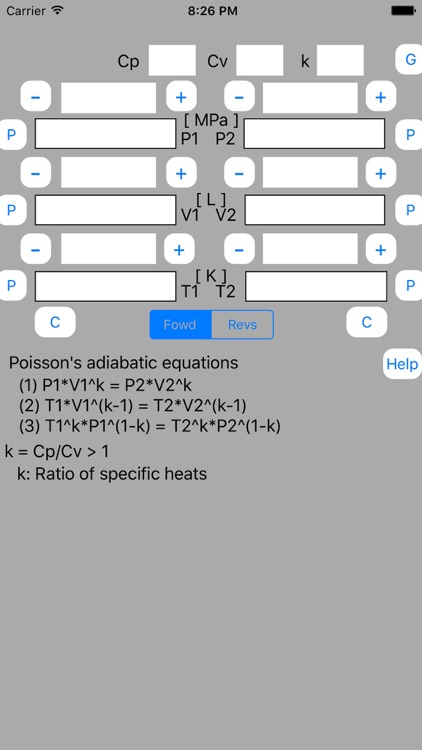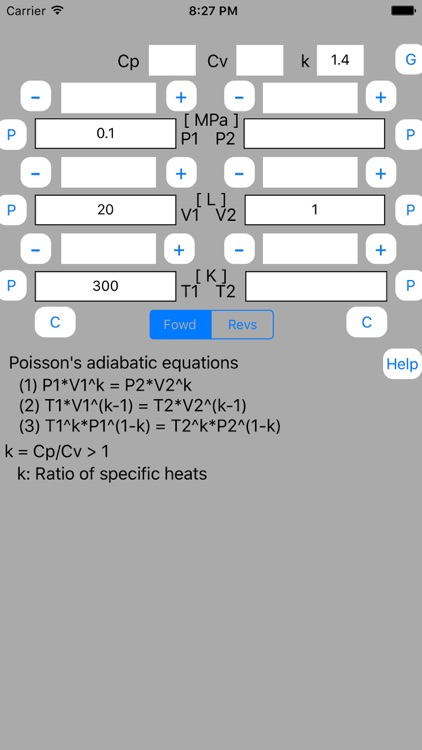Adiabatic process is a process in which heat exchange does not take place between a　thermodynamic system and its surroundings. Behavior of reversible adiabatic process is described by Poisson's equations. Adiabatic Calculator mini is a software that solves the Poisson's equations. This Adiabatic Calculator mini is a subset of iPad Adiabatic Calculator software. Pressure unit and temperature unit are fixed to [MPa] and Kelvin temperature [K].### App Details

Version
1.03
Rating
NA
Size
0Mb
Genre
Utilities Education
Last updated
November 23, 2016
Release date
November 20, 2015

### App Screenshots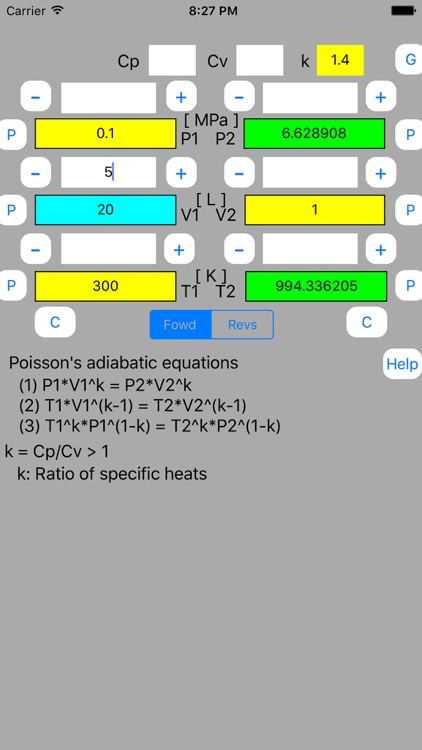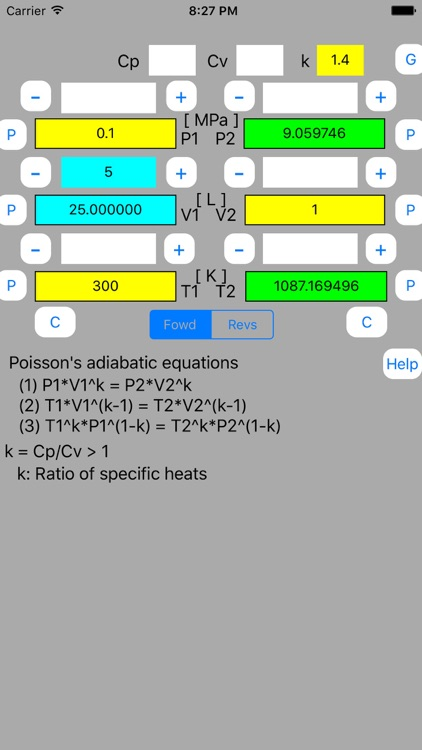### App Store Description

Adiabatic process is a process in which heat exchange does not take place between a　thermodynamic system and its surroundings. Behavior of reversible adiabatic process is described by Poisson's equations. Adiabatic Calculator mini is a software that solves the Poisson's equations. This Adiabatic Calculator mini is a subset of iPad Adiabatic Calculator software. Pressure unit and temperature unit are fixed to [MPa] and Kelvin temperature [K].
It's possible to change one of input data one after another. Thus it's possible to get output data which correspond to the changed input data.

(Example)
There is air of 1 atmosphere ( = 0.1[MPa]) and 300[K]. When volume of the air is adiabatically compressed into 1/20, how do the temperature and the pressure change?
When the volume is compressed into 1/25, 1/30, and 1/35, how do they change?
Assume that ratio of specific heats of the air is 1.4

This software calculates output data based on one pair data of initial and final state. Ratio of the pair data is used for the calculation.
In this example, pair data is initial and final volumes
Enter pair data as follows.
V1 = 20[L], Vo12 = 1[L]
Enter other known data as follows.
P1 = 0.1[MPa]
T1 = 300[K].
Enter ratio of specific heats as follows.
k = 1.4

To execute calculation, P button of the pair data is touched out. There are initial side and final side P buttons for the pair data. Which side button to select depends on calculation mode.
In this example, we want to get P2 and T2 data that correspond to changed V1 data. Output data is opposite side to the input data. In this case, set calculation mode to Fowd mode.
Touch out V1_P button.

We obtain 6.63[MPa] and 994.3[K] as the final pressure and the final temperature , respectively.

Next enter increment data as follows.
Delta = 5[L]

Touch out + button.

For the volume compression into 1/25, we obtain 9.1[MPa] and 1087[K] as the final pressure and the final temperature.
Next touch out + button. We can obtain the next output data.
By use of + or - button, it's possible to change one of input data one after another and to get output data which corresponds to the changed input data.

Disclaimer:
AppAdvice does not own this application and only provides images and links contained in the iTunes Search API, to help our users find the best apps to download. If you are the developer of this app and would like your information removed, please send a request to [email protected] and your information will be removed.